Courses

# Ex 1.3 NCERT Solutions - Real Numbers Class 10 Notes | EduRev

## Class 10 : Ex 1.3 NCERT Solutions - Real Numbers Class 10 Notes | EduRev

The document Ex 1.3 NCERT Solutions - Real Numbers Class 10 Notes | EduRev is a part of the Class 10 Course Mathematics (Maths) Class 10.
All you need of Class 10 at this link: Class 10

Exercise 1.3
Ques 1: Prove that √5 is irrational.

Sol: Let √5 be a rational number.
∴ We have to find two integers a and b (where, b ≠ 0 and a and b are coprime) such that
a/b = √5
⇒ a = √5.b      ...(1)
Squaring both sides, we have
a2 = 5b2
∴ 5 divides a2
⇒ 5 divides a   ...(2)
[∵ a prime number ‘p’ divides a2 then ‘p’ divides ‘a’, where ‘a’ is positive integer.]
∴ a  =  5c,  where c is an integer.
∴ Putting a = 5c in (1), we have
5c = √5. b
or (5c)2 =  5b2
⇒ 25c2 = 5b2
⇒ 5c= b2
⇒ 5 divides b2
⇒ 5 divides b            ...(3)
From (2) and (3)
a and b have at least 5 as a common factor.
i.e., a and b are not coprime.
∴ Our supposition that √5 is rational is wrong.
Hence, √5 is irrational.

Ques 2: Prove that 3+2√5 is irrational.
Sol: Let 3+2√5 is rational.
∴ We can find two coprime integers ‘a’ and ‘b’ such that
[3+2√5] =  a/b,  where  b ≠ 0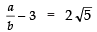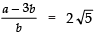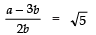∵ a and b are integers,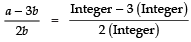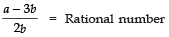⇒ (1) is a rational
⇒ √5 is a rational
But this contradicts the fact that √5 is irrational.
∴ Our supposition is wrong.
3+2√5  is an irrational.

Ques 3: Prove that the following are irrationals:
(i) 1/√2
(ii) 7√5
(iii) 6+√2

Sol: (i) We have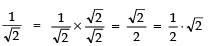Let 1/√2  be rational, and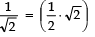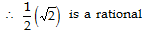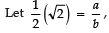such that ‘a’ and ‘b’ are coprime integers and b ≠ 0.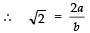...(1)
since, the division of two integers is rational.
∴ 2a/b is a rational.
From (1), √2 is a rational number which contradicts the fact that √2 is irrational.
∴ Our assumption is wrong.
Thus, 1√2 is irrational.
(ii) Let us suppose that 7√5 is rational.
Let there be two coprime integers ‘a’ and ‘b’.
such that 7√5 =  a/b , where b ≠ 0
Now, = 7√5 = a/b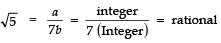⇒ √5 is a rational
This contradicts the fact that √5 is irrational.
∴ We conclude that 7√5 is irrational.
(iii) Let us suppose that 6+√2 is rational.
∴ We can find two coprime integers ‘a’ and ‘b’ (b ≠ 0), such that
6+√2  = a/b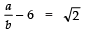or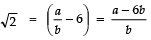...(1)
∵ a and b are integers,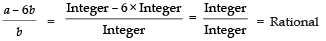[∵ subtraction of integers is also an integer]
[∵ Division of two integers is a rational number]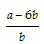is a rational number.
From (1), √2 is a rational number, which contradicts the fact that √2 is an irrational number.
∴ Our supposition is wrong.
⇒ 6+ √2 is an irrational number.

Revisiting Rational Numbers and Their Decimal Expansion
Remember:
I. The decimal expansion of every rational number is either terminating or non terminating and repeating.
II. For any rational number p/q having terminating decimal representation, the prime factorisation of ‘q’ is of the form 2n 5m, where n and m are non-negative integers.
III. For any rational number p/q, if the prime factorisation of q is of the form 25m, where n and m are non-negative integers, then its decimal representation is terminating.
IV. For any rational number p/q, if the prime factorisation of q is not of the form 2n 5m, where m and n are non-negative integers, then its decimal representation is non terminating and repeating.

Offer running on EduRev: Apply code STAYHOME200 to get INR 200 off on our premium plan EduRev Infinity!

## Mathematics (Maths) Class 10

178 videos|268 docs|103 tests

,

,

,

,

,

,

,

,

,

,

,

,

,

,

,

,

,

,

,

,

,

;